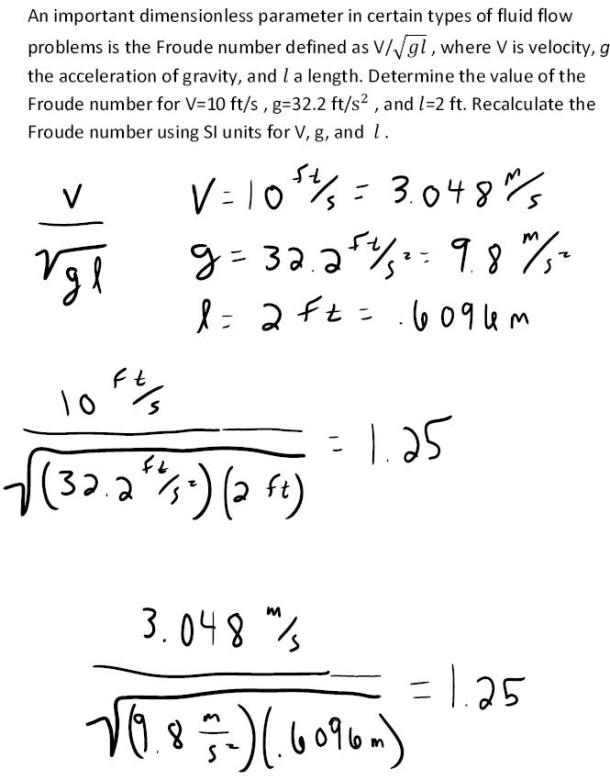fluid mechanics math problems engineering equations fluid mechanics basic mechanics school homework engineering math fluids formulas fluid mechanics problems fluid mechanics problem solutions to fluids problems full solution fluid mechanics engineering fluids problem solution fluid mechanics math problems engineering equations fluid mechanics basic mechanics school homework engineering math fluid mechanics formulas fluid problems fluid mechanics problem solutions to fluid mechanics problems full solution fluid mechanics engineering fluid mechanics problem solution
fluid mechanics math problems engineering equations fluid mechanics basic mechanics school homework engineering math fluids formulas fluid mechanics problems fluid mechanics problem solutions to fluids problems full solution fluid mechanics engineering fluids problem solution fluid mechanics math problems engineering equations fluid mechanics basic mechanics school homework engineering math fluid mechanics formulas fluid problems fluid mechanics problem solutions to fluid mechanics problems full solution fluid mechanics engineering fluid mechanics problem solution
Highalphabet Home Page fluid mechanics solutions fluids math solved Fluid Mechanics Page 1
An important dimensionless parameter in certain types of fluid flow problems is the Froude number defined as V/(gl)^(1/2), where V is velocity, g the acceleration of gravity, and l a length. Determine the value of the Froude number for V=10 ft/s, g=32.2 ft/s^2, and l=2 ft. Recalculate the Froude number using SI units for V,G, and l.An important dimensionless parameter in certain types of fluid flow problems is the Froude number defined as V/(gl)^(1/2), where V is velocity, g the acceleration of gravity, and l a length. Determine the value of the Froude number for V=10 ft/s, g=32.2 ft/s^2, and l=2 ft. Recalculate the Froude number using SI units for V,G, and l.